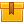# Ohm's Law (A8201)

## Prerequisites

This lesson is designed so that no prior knowledge is required.

## Description

This lesson shows and explains how a basic DC electrical circuit operates and how voltage, current, and resistance behave in a series circuit, a parallel circuit, and a series parallel circuit. Procedures for using Ohm??s Law to calculate voltage, current, and resistance in a circuit are also provided.

## Objectives

Define electricity. Describe how a simple electrical circuit operates. Use Ohm??s Law to calculate voltage, current, and resistance in any kind of circuit.

## ContentThis lesson shows and explains how a basic DC electrical circuit
operates and how voltage, current, and resistance behave in a series
circuit, a parallel circuit, and a series parallel circuit.  Procedures
for using Ohm’s Law to calculate voltage, current, and resistance in a
circuit are also provided.

•Ohm's Law

0
0 Reviews